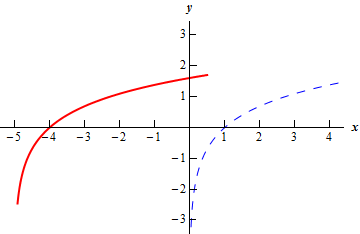Paul's Online Notes
Home / Algebra / Exponential and Logarithm Functions / Logarithm Functions
Show Mobile Notice Show All Notes Hide All Notes
Mobile Notice
You appear to be on a device with a "narrow" screen width (i.e. you are probably on a mobile phone). Due to the nature of the mathematics on this site it is best views in landscape mode. If your device is not in landscape mode many of the equations will run off the side of your device (should be able to scroll to see them) and some of the menu items will be cut off due to the narrow screen width.

### Section 6.2 : Logarithm Functions

22. Sketch the graph of $$g\left( x \right) = \ln \left( {x + 5} \right)$$ .

Show Solution

For this problem all we need to do is recall the Transformations section from a couple of chapters ago. Using the “base” function of $$f\left( x \right) = \ln \left( x \right)$$ the function for this part can be written as,

$g\left( x \right) = \ln \left( {x + 5} \right) = f\left( {x + 5} \right)$

Therefore, the graph for this part is just the graph of $$f\left( x \right)$$ shifted left by 5.

The graph of this function is shown below. The blue dashed line is the “base” function, $$f\left( x \right)$$, and the red solid line is the graph for this part, $$g\left( x \right)$$.Do not get excited about the fact that we plugged negative values of $$x$$ into the function! The problem with negative values is not the values we plug into a logarithm. Instead the problem with negative values is when we go to evaluate the logarithm.

It is perfectly fine to plug negative values into a logarithm as long as we don’t end up actually evaluating a negative number. So, in this case we can see that as long as we require $$x > - 5$$ then $$x + 5 > 0$$ and so those are acceptable values of $$x$$ to plug in since we aren’t going to evaluate negative number in the logarithm.

Note however that we do have avoid $$x < - 5$$ since that would mean evaluating logarithms at negative numbers.